# Project - Selecting Content for Data Science Company¶

In this project, we will try to answer the question: "What do people want to learn in data science", and in so doing evaluate the available content for our company which deals with data science education.

Scenario

We're working for a company that creates data science content, be it books, online articles, videos or interactive text-based platforms. We're tasked with figuring out what is best content to write about. We realize that if we wanted to figure out what programming content to write, we could consult Stack Overflow (a question and answer website about programming) and see what kind of content is more popular. After investigating Stack Overflow in depth, we find out that it is part of a question and answer website network called Stack Exchange.

### Stack Exchange¶

Stack Exchange hosts sites on a multitude of fields and subjects, including mathematics, physics, philosophy, and data science! Here's a sample of the most popular sites: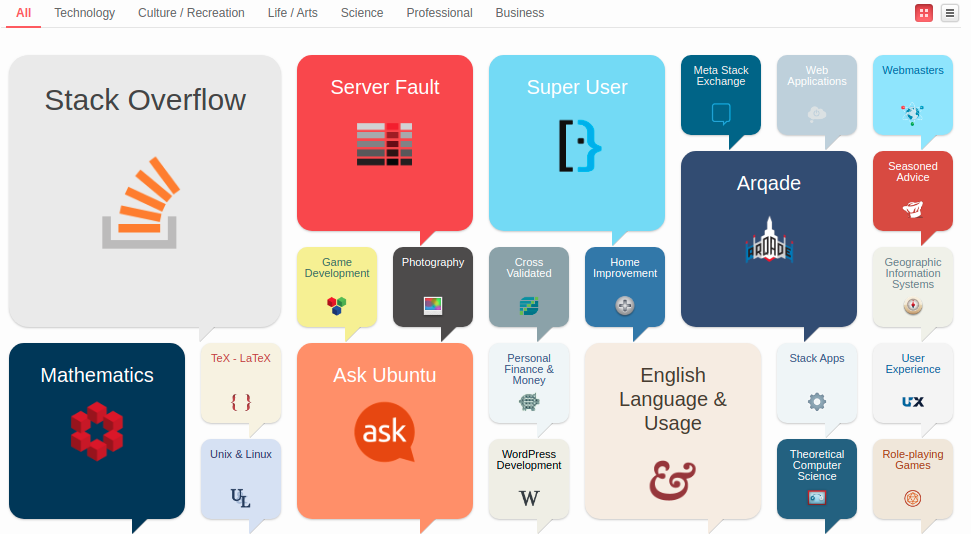Stack Exchange employs a reputation award system for its questions and answers. Each post — each question/answer — is a post that is subject to upvotes and downvotes. This ensures that good posts are easily identifiable. More details are available on this tour

Being a multidisciplinary field, there a few Stack Exchange websites there are relevant to our goal here:

And if we want to include Data Engineering, we can also consider:

At the time of writing, in terms of users Data Science Stack Exchange (DSSE) is among top 40 sites with 85K users, however, in terms of unanswered questions it is among bottom 7 with 64% unanswered questions. Further statistics are available here. This makes it quite attractive for exploring data science content.

### Data Science Stack Exchange (DSSE)¶

The site is organized into following sections:

• Home: Displays the top questions and contains a side bar menu with links to other sections of the site.

• [Questions]: Features all questions which can be filtered by No answers and No accepted answers. Currently, approximately 25,000 questions are featured of which over 15,000 are unanswered or with no accepted answer (63%).

• Tags are key words which organize questions as topics and facilitate search.

• Users features the data on users. Users with highest reputation are featured before the others. Users can be searched and filtered by user name, reputation, New user etc.

• Unanswered features unanswered questions which can be filtered by votes, tags and newest.

The footer menu has links to Tour and Help sections along with links to other Stack Exchange sites and features.

Questions and Answers

Data Science Stack Exchange(DSSE) is a question and answer site for Data science professionals, Machine Learning specialists, and those interested in learning more about the field.The method of asking questions, getting answers, and getting promoted to higher levels is given here:

• The site is all about getting answers. It's not a discussion forum. There's no chit-chat.
• Good answers are voted up and rise to the top. The best answers show up first so that they are always easy to find.
• The person who asked can mark one answer as "accepted". Accepting doesn't mean it's the best answer, it just means that it worked for the person who asked.
• Focus on questions about an actual problem you have faced. Include details about what you have tried and exactly what you are trying to do.
• Avoid questions that are primarily opinion-based, or that are likely to generate discussion rather than answers. Questions that need improvement may be closed until someone fixes them.
• All questions are tagged with their subject areas. Each can have up to 5 tags. We can click any tag to see a list of questions with that tag, or go to the tag list to browse for topics 0f interest.
• User reputation score goes up when others vote up on their questions, answers and edits.
• User Privileges are granted based on reputation score.

### Getting Data¶

Stack Exchange sites have a number of convenient ways of getting data:

• Scraping : We can scrape a page for relevant information.

In this case, we will be retrieving tags on the first page of the tags link

In [ ]:
import requests
# request data from "https://datascience.stackexchange.com/tags"
response = requests.get("https://datascience.stackexchange.com/tags")

content = response.content

from bs4 import BeautifulSoup

# Initialize the parser, and pass in the content we grabbed earlier.

parser = BeautifulSoup(content, 'html.parser')

tag = parser.select(".post-tag")

tags_scraping = []

for i in range(0,36):
tag_text = tag[i].text
tags_scraping.append(tag_text)
print(tags_scraping)

['machine-learning', 'python', 'neural-network', 'deep-learning', 'classification', 'keras', 'scikit-learn', 'tensorflow', 'nlp', 'r', 'time-series', 'dataset', 'regression', 'data-mining', 'clustering', 'cnn', 'predictive-modeling', 'pandas', 'lstm', 'statistics', 'feature-selection', 'data', 'random-forest', 'image-classification', 'decision-trees', 'linear-regression', 'text-mining', 'data-cleaning', 'visualization', 'reinforcement-learning', 'rnn', 'xgboost', 'logistic-regression', 'convnet', 'bigdata', 'svm']


API

Obviously, scraping is a tedious process and getting even the names of tags will involve a lot of coding.

Next, we can try the Stack Exchange API

First, we register the app for access token

• App: Py Lesson
• Get client_id = 18238
• redirect_uri = https://stackoverflow.com/oauth/login_success
In [ ]:
# access token requested
import requests
import requests.auth

response = requests.post("https://stackoverflow.com/oauth/dialog?client_id=18238&redirect_uri=https://stackoverflow.com/oauth/login_success")

print(response.status_code)

200

In [ ]:
# access_token is only required for increased quota, write and access to private info
## Following access token is now expired

headers = {'access_token': '6QkGLWxpVNzS6XWzfOFXGw))', 'key': 'LCyb3nlOflFZqImiVbfZog((' }

response = requests.get("https://api.stackexchange.com/2.2/tags?page=1&pagesize=36&order=desc&sort=popular&site=datascience", headers=headers)
tags = response.json()

In [ ]:
# Parse the json for extracting names of tags on page-1
import pandas as pd

tags_list = tags['items']

tags_df = pd.DataFrame(tags_list)

tags_api = tags_df["name"]
print(tags_api)

0           machine-learning
1                     python
2             neural-network
3              deep-learning
4             classification
5                      keras
6               scikit-learn
7                 tensorflow
8                        nlp
9                          r
10               time-series
11                   dataset
12                regression
13               data-mining
14                clustering
15                       cnn
16       predictive-modeling
17                    pandas
18                      lstm
19                statistics
20         feature-selection
21                      data
22             random-forest
23      image-classification
24            decision-trees
25         linear-regression
26               text-mining
27             data-cleaning
28             visualization
29    reinforcement-learning
30                       rnn
31                   xgboost
32       logistic-regression
33                   convnet
34                   bigdata
35                       svm
Name: name, dtype: object

In [ ]:
# Check whether tag list obtained from scraping and from api are equal
tags_api = list(tags_df["name"])

equal = tags_api==tags_scraping
print(equal) # Are equal

True


Stack Exchange Data Explorer (SEDE)

SEDE is an open source tool for running arbitrary queries against public data from the Stack Exchange network. Features include collaborative query editing for all graduated and public beta Stack Exchange sites.

The data is updated early every Sunday morning around 3:00 UTC.

Apart from web-scraping and use of API, SEDE provides access to the database and entertains T-SQL queries.

Following query run at SEDE gives the same results as obtained from web-scraping and API.

SELECT TOP 36
TagName, COUNT
FROM Tags
ORDER BY COUNT DESC;

The query results can be downloaded as a csv file, and a permalink can also be created to the query (as done above). We will now compare results from the query with those of web_scraping and API.

In [ ]:
import pandas as pd
query = pd.read_csv("top_36_tags.csv")
tags_query = query["TagName"]
tags_query = list(tags_query)
print(tags_query)

['machine-learning', 'python', 'neural-network', 'deep-learning', 'classification', 'keras', 'scikit-learn', 'tensorflow', 'nlp', 'r', 'time-series', 'dataset', 'regression', 'data-mining', 'clustering', 'cnn', 'predictive-modeling', 'pandas', 'lstm', 'statistics', 'feature-selection', 'data', 'random-forest', 'image-classification', 'decision-trees', 'linear-regression', 'text-mining', 'data-cleaning', 'visualization', 'reinforcement-learning', 'rnn', 'xgboost', 'logistic-regression', 'convnet', 'bigdata', 'svm']

In [ ]:
# Check whether these results are same as those obtained from web-scraping and API

equal = tags_api==tags_scraping==tags_query

print(equal) # All results are equal

True

In [ ]:
# Determine top ten popular topics from tags
top_ten = query[:10]
print(top_ten)

            TagName  COUNT
0  machine-learning   7879
1            python   4612
2    neural-network   3304
3     deep-learning   3214
4    classification   2182
5             keras   2058
6      scikit-learn   1542
7        tensorflow   1475
8               nlp   1393
9                 r   1224


### Getting Posts Data from SEDE¶

Above, we can see that maximum questions are contained in about top 10 or so tags. Same content (top 36 tags) is displayed on the tags home page Now we will focus on the Posts table in the database SEDE to create a database for a year (2019) and carry out a more granular analysis.

We will run a query against the SEDE DSSE database that extracts the following columns for all the questions in 2019: Id: An identification number for the post. PostTypeId: An identification number for the type of post. CreationDate: The date and time of creation of the post. Score: The post's score. ViewCount: How many times the post was viewed. Tags: What tags were used. AnswerCount: How many answers the question got (only applicable to question posts). FavoriteCount: How many times the question was favored (only applicable to question posts).

Using the API in Tandem

We will use the API in tandem with query in order to compare some of our results.

In [ ]:
# Find total number of questions in 2019 using API

response = requests.get("https://api.stackexchange.com/2.2/questions?pagesize=100&fromdate=1546300800&todate=1577750400&order=desc&sort=creation&site=stackoverflow&filter=!5-dmZUPsa(aVhP2rEGYktwXr6kydXo7VO6LStu", headers=headers)
questions_api = response.json()

questions_list = questions_api['items']
questions_100 = pd.DataFrame(questions_list)
print(questions_100.info())

<class 'pandas.core.frame.DataFrame'>
RangeIndex: 100 entries, 0 to 99
Data columns (total 8 columns):
answer_count      100 non-null int64
creation_date     100 non-null int64
favorite_count    100 non-null int64
question_id       100 non-null int64
score             100 non-null int64
tags              100 non-null object
title             100 non-null object
view_count        100 non-null int64
dtypes: int64(6), object(2)
memory usage: 6.3+ KB
None


# Find total number of questions from query¶

We can see from above that the api is limited to returning 100 results per page and getting all the data would need running a loop several times consuming computational resources. We will, therefore, get the complete data from the database running following query

SELECT Id, CreationDate,
Score, ViewCount, Tags,
AnswerCount, FavoriteCount
FROM posts
WHERE PostTypeId = 1 AND YEAR(CreationDate) = 2019;

Note that of the various post types Post TypeID for "questions" is 1.

We download the results as 2019_questions.csv from SEDE.

In [ ]:
# Convert the results into dataframe

questions = pd.read_csv("2019_questions.csv", parse_dates=["CreationDate"])

print(questions.info())

<class 'pandas.core.frame.DataFrame'>
RangeIndex: 7948 entries, 0 to 7947
Data columns (total 7 columns):
Id               7948 non-null int64
CreationDate     7948 non-null datetime64[ns]
Score            7948 non-null int64
ViewCount        7948 non-null int64
Tags             7948 non-null object
AnswerCount      7948 non-null int64
FavoriteCount    1517 non-null float64
dtypes: datetime64[ns](1), float64(1), int64(4), object(1)
memory usage: 434.7+ KB
None


### Data Cleaning¶

We want to eventually focus on the tags column as well as other popularity, so we will carry out following data-cleaning steps:

• Fill missing values in FavoriteCount with 0 as the missing values indicate that the question was not voted upon.
• Convert FavoriteCount into int
• Convert Tags string into a more readable format
In [ ]:
# Fill in missing values for the "FavoriteCount" column

questions.fillna(0, inplace=True)
questions["FavoriteCount"] = questions["FavoriteCount"].astype(int)

print(questions.info())

<class 'pandas.core.frame.DataFrame'>
RangeIndex: 7948 entries, 0 to 7947
Data columns (total 7 columns):
Id               7948 non-null int64
CreationDate     7948 non-null datetime64[ns]
Score            7948 non-null int64
ViewCount        7948 non-null int64
Tags             7948 non-null object
AnswerCount      7948 non-null int64
FavoriteCount    7948 non-null int64
dtypes: datetime64[ns](1), int64(5), object(1)
memory usage: 434.7+ KB
None

In [ ]:
# Convert format of tags string

print(questions["Tags"].sample(5))

508     <deep-learning><numpy><plotting><matplotlib><i...
6198    <machine-learning><data-mining><data><algorith...
5562                                               <tsne>
2681                                         <statistics>
1936    <neural-network><deep-learning><lstm><reinforc...
Name: Tags, dtype: object

In [ ]:
questions["Tags"] = questions["Tags"].str.replace('^<|>$','').str.split('><') print(questions["Tags"].sample(5))  5582 [python, statistics, data, ipython] 5807 [named-entity-recognition, tfidf] 2536 [machine-learning, neural-network, lstm, recur... 6921 [deep-learning, tensorflow, pytorch, transform... 3453 [python, image-classification, computer-vision... Name: Tags, dtype: object  ### Most Used and Most Viewed Tags¶ We will focus on Tags to determine: • Count how many times each tag was used. • Count how many times each tag was viewed. • Create visualizations for the top tags of each of the above results. In [ ]: print(questions.sample(5))   Id CreationDate Score ViewCount \ 1225 56141 2019-07-22 09:26:29 0 24 5557 63108 2019-11-13 18:27:55 1 39 762 45343 2019-02-10 17:13:39 1 596 4707 53839 2019-06-15 08:05:34 6 723 2635 61749 2019-10-15 08:21:38 0 21 Tags AnswerCount \ 1225 [machine-learning, neural-network, deep-learni... 0 5557 [machine-learning, python, keras, image-prepro... 2 762 [machine-learning, neural-network, deep-learni... 0 4707 [bigdata, data, xgboost] 3 2635 [time-series] 1 FavoriteCount 1225 0 5557 0 762 0 4707 3 2635 0  In [ ]: tag_no = {} for tags in questions["Tags"]: for tag in tags: if tag not in tag_no: tag_no[tag] = 1 else: tag_no[tag] += 1  In [ ]: tag_no = pd.DataFrame.from_dict(data=tag_no, orient="index") tag_no.rename(columns={0: "No"}, inplace=True) most_used=tag_no.sort_values(by='No', ascending=False, axis=0) most_used.head(20)  Out[ ]: No machine-learning 2443 python 1652 deep-learning 1082 neural-network 960 keras 841 classification 629 tensorflow 515 scikit-learn 491 nlp 450 cnn 439 time-series 405 lstm 341 pandas 340 regression 316 dataset 301 clustering 249 r 243 predictive-modeling 242 statistics 211 machine-learning-model 206 ### Using SEDE Query¶ We can use the SEDE Query to obtain the same results as above. SELECT TagName, COUNT(TagName) FROM Posts AS p INNER JOIN PostTags AS pt ON pt.PostId=p.id INNER JOIN Tags AS t ON t.id=pt.TagId WHERE PostTypeId = 1 AND YEAR(CreationDate) = 2019 GROUP BY TagName ORDER BY COUNT(TagName) DESC; In [ ]: tags_2019 = pd.read_csv("tags_2019.csv") tags_2019.rename(columns={"Unnamed: 1": "No"}, inplace=True) print(tags_2019.head(20))   TagName No 0 machine-learning 2443 1 python 1652 2 deep-learning 1082 3 neural-network 960 4 keras 841 5 classification 629 6 tensorflow 515 7 scikit-learn 491 8 nlp 450 9 cnn 439 10 time-series 405 11 lstm 341 12 pandas 340 13 regression 316 14 dataset 301 15 clustering 249 16 r 243 17 predictive-modeling 242 18 statistics 211 19 machine-learning-model 206  In [ ]: # Results obtained from query and dataset are equal equal = list(most_used["No"]) == list(tags_2019["No"]) print(equal)  True  In [ ]: # Determining Viewcounts for Tags in 2019 view_no = {} for index, row in questions.iterrows(): for tag in row['Tags']: if tag not in view_no: view_no[tag] = row['ViewCount'] else: view_no[tag] += row['ViewCount']  In [ ]: view_no = pd.DataFrame.from_dict(data=view_no, orient="index") view_no.rename(columns={0: "No"}, inplace=True) most_viewed=view_no.sort_values(by='No', ascending=False, axis=0).head(20) most_viewed  Out[ ]: No python 1196833 machine-learning 773935 keras 544628 pandas 527831 deep-learning 453475 neural-network 380012 scikit-learn 280534 tensorflow 257580 classification 209486 dataframe 203979 nlp 152420 cnn 151930 lstm 143433 time-series 130870 numpy 116095 regression 94623 csv 88767 pytorch 88365 dataset 83026 seaborn 74060 ### Using SEDE Query¶ We can use the SEDE Query to obtain the same results as above. SELECT TagName, SUM(ViewCount) FROM Posts AS p INNER JOIN PostTags AS pt ON pt.PostId=p.id INNER JOIN Tags AS t ON t.id=pt.TagId WHERE (PostTypeId = 1 AND YEAR(CreationDate) = 2019) GROUP BY TagName ORDER BY 2 DESC; In [ ]: views_2019 = pd.read_csv("views_2019.csv") views_2019.rename(columns={"Unnamed: 1": "No"}, inplace=True) print(views_2019.head(20))   TagName No 0 python 1196833 1 machine-learning 773935 2 keras 544628 3 pandas 527831 4 deep-learning 453475 5 neural-network 380012 6 scikit-learn 280534 7 tensorflow 257580 8 classification 209486 9 dataframe 203979 10 nlp 152420 11 cnn 151930 12 lstm 143433 13 time-series 130870 14 numpy 116095 15 regression 94623 16 csv 88767 17 pytorch 88365 18 dataset 83026 19 seaborn 74060  In [ ]: # Results obtained from query and dataset are equal equal = list(most_viewed["No"]) == list(views_2019["No"].head(20)) print(equal)  True  In [ ]: import matplotlib.pyplot as plt %matplotlib inline most_used.plot(kind="barh", figsize=(16,8),logx=True) #log scaling on x axis due to very large numbers  Out[ ]: <matplotlib.axes._subplots.AxesSubplot at 0x7f17fc305860>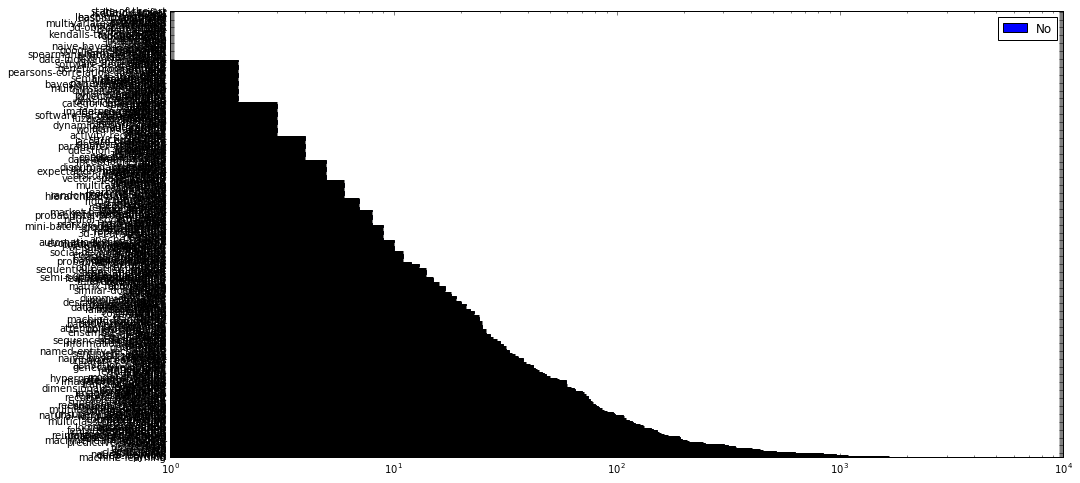In [ ]: most_viewed.plot(kind="barh", figsize=(16,8),logx=True)#log scaling on x axis due to very large numbers  Out[ ]: <matplotlib.axes._subplots.AxesSubplot at 0x7f17f9bdd160>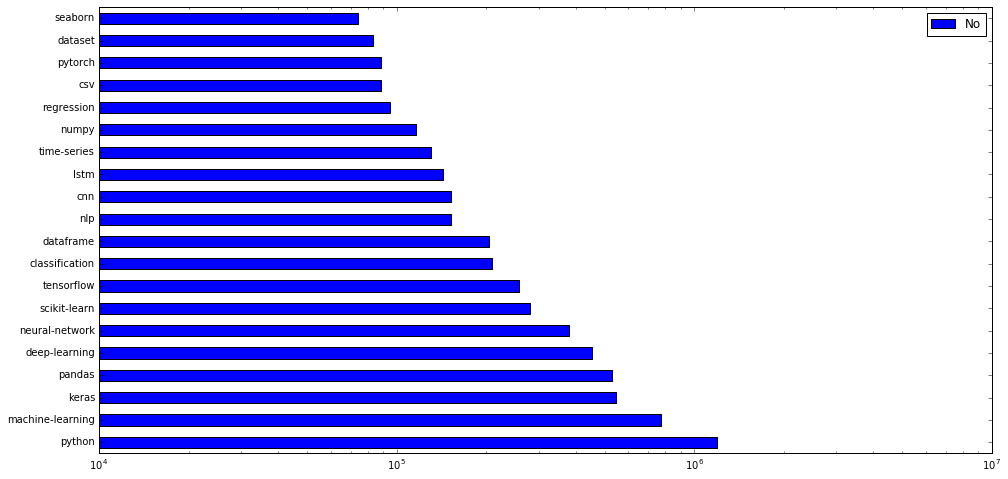In [ ]: fig, axes = plt.subplots(1, 2) fig.set_size_inches((28, 12)) #log scaling on x axis due to very large numbers most_used.plot(kind="barh", ax=axes, subplots=True, logx=True) most_viewed.plot(kind="barh", ax=axes, subplots=True, logx=True)  Out[ ]: array([<matplotlib.axes._subplots.AxesSubplot object at 0x7f17f856f5f8>], dtype=object)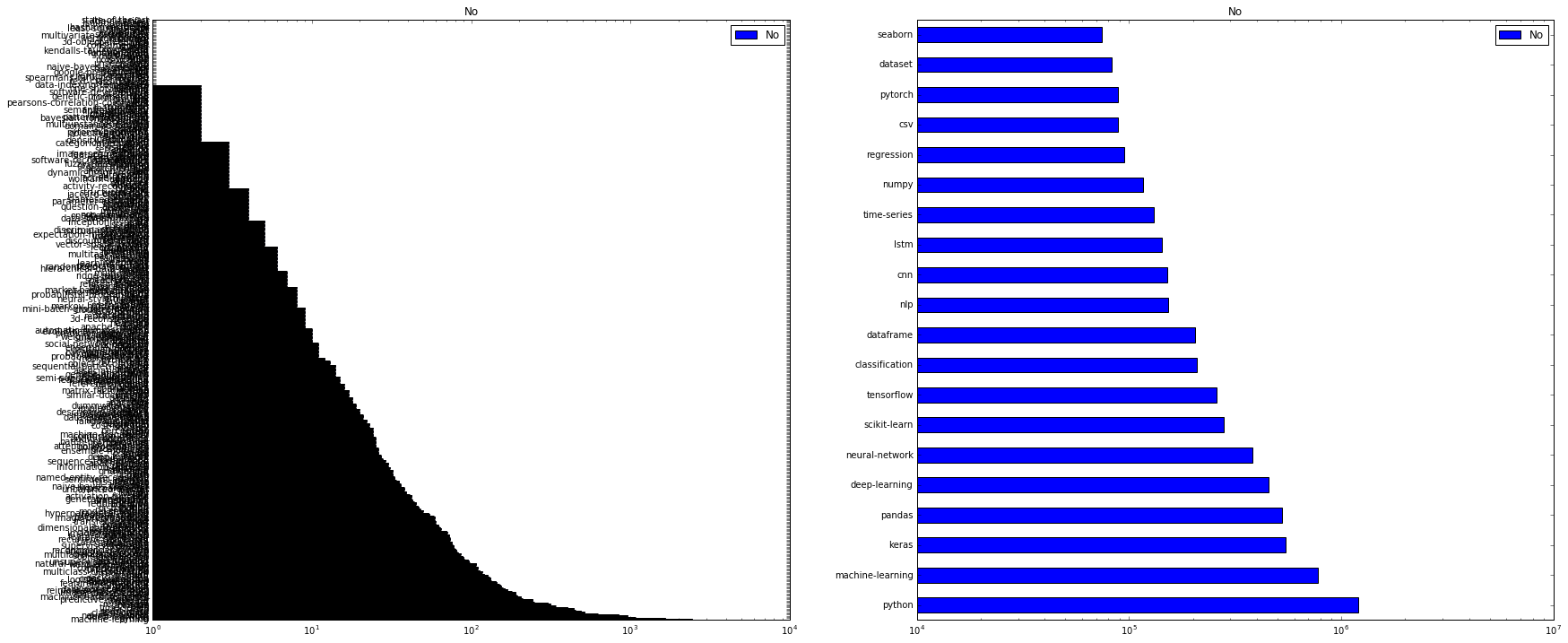### Relations Between Tags¶ If we want to see what tags are in most_used, but not in most_viewed, We can identify them by the missing values in ViewCount. Similarly if we want to know which tags are in the latter, but not the former we can use a similar approach. In [ ]: in_used = pd.merge(most_used, most_viewed, how="left", left_index=True, right_index=True) print(in_used)   No_x No_y machine-learning 2443 773935.0 python 1652 1196833.0 deep-learning 1082 453475.0 neural-network 960 380012.0 keras 841 544628.0 classification 629 209486.0 tensorflow 515 257580.0 scikit-learn 491 280534.0 nlp 450 152420.0 cnn 439 151930.0 time-series 405 130870.0 lstm 341 143433.0 pandas 340 527831.0 regression 316 94623.0 dataset 301 83026.0 clustering 249 NaN r 243 NaN predictive-modeling 242 NaN statistics 211 NaN machine-learning-model 206 NaN data-mining 196 NaN data 192 NaN feature-selection 191 NaN image-classification 189 NaN reinforcement-learning 180 NaN data-science-model 169 NaN linear-regression 163 NaN xgboost 158 NaN pytorch 155 88365.0 loss-function 152 NaN ... ... ... mongodb 1 NaN google-cloud 1 NaN functional-api 1 NaN kendalls-tau-coefficient 1 NaN stata 1 NaN bayes-error 1 NaN corpus 1 NaN spyder 1 NaN consumerweb 1 NaN lime 1 NaN open-source 1 NaN 3d-object-detection 1 NaN gru 1 NaN usecase 1 NaN version-control 1 NaN manifold 1 NaN multivariate-distribution 1 NaN statsmodels 1 NaN community 1 NaN adaboost 1 NaN .net 1 NaN redshift 1 NaN least-squares-svm 1 NaN hashingvectorizer 1 NaN wikipedia 1 NaN hog 1 NaN nl2sql 1 NaN isolation-forest 1 NaN lda-classifier 1 NaN state-of-the-art 1 NaN [503 rows x 2 columns]  In [ ]: pd.merge(most_used, most_viewed, how="right", left_index=True, right_index=True)  Out[ ]: No_x No_y python 1652 1196833 machine-learning 2443 773935 keras 841 544628 pandas 340 527831 deep-learning 1082 453475 neural-network 960 380012 scikit-learn 491 280534 tensorflow 515 257580 classification 629 209486 dataframe 76 203979 nlp 450 152420 cnn 439 151930 lstm 341 143433 time-series 405 130870 numpy 110 116095 regression 316 94623 csv 24 88767 pytorch 155 88365 dataset 301 83026 seaborn 35 74060 • clustering, r, predictive-modeling, statistics and machine-learning-model are in most_used but not in most_viewed. • dataframe, numpy, csv, pytorch and seaborn are in most_viewed but not in most-used. Simpler Approach using Sets A far simpler appraoch is to use set methods intersection and difference • As is obvious below, it gives the same results as above. In [ ]: set(most_used.index).intersection(set(most_viewed.index))  Out[ ]: {'classification', 'cnn', 'csv', 'dataframe', 'dataset', 'deep-learning', 'keras', 'lstm', 'machine-learning', 'neural-network', 'nlp', 'numpy', 'pandas', 'python', 'pytorch', 'regression', 'scikit-learn', 'seaborn', 'tensorflow', 'time-series'} In [ ]: set(most_used.index).difference(set(most_viewed.index))  Out[ ]: {'.net', '3d-object-detection', '3d-reconstruction', 'ab-test', 'accuracy', 'activation-function', 'active-learning', 'activity-recognition', 'actor-critic', 'adaboost', 'aggregation', 'ai', 'alex-net', 'algorithms', 'allennlp', 'anaconda', 'ann', 'annotation', 'anomaly', 'anomaly-detection', 'anonymization', 'anova', 'apache-hadoop', 'apache-spark', 'arima', 'association-rules', 'attention-mechanism', 'auc', 'audio-recognition', 'autoencoder', 'automatic-summarization', 'automation', 'automl', 'aws', 'aws-lambda', 'azure-ml', 'backpropagation', 'batch-normalization', 'bayes-error', 'bayesian', 'bayesian-networks', 'bayesian-nonparametric', 'beginner', 'bert', 'bias', 'bigdata', 'binary', 'bioinformatics', 'books', 'boosting', 'c', 'c++', 'caffe', 'career', 'categorical-data', 'categorical-encoding', 'categories', 'causalimpact', 'chatbot', 'churn', 'class-imbalance', 'classifier', 'cloud', 'cloud-computing', 'clustering', 'clusters', 'code', 'colab', 'collinearity', 'community', 'competitions', 'computer-vision', 'confusion-matrix', 'consumerweb', 'convergence', 'convnet', 'convolution', 'corpus', 'correlation', 'cosine-distance', 'cost-function', 'counts', 'coursera', 'crawling', 'cross-validation', 'data', 'data-analysis', 'data-augmentation', 'data-cleaning', 'data-formats', 'data-imputation', 'data-indexing-techniques', 'data-leakage', 'data-mining', 'data-product', 'data-science-model', 'data-stream-mining', 'data-wrangling', 'data.table', 'databases', 'dbscan', 'decision-trees', 'deep-network', 'deepmind', 'definitions', 'density-estimation', 'derivation', 'descriptive-statistics', 'difference', 'dimensionality-reduction', 'dirichlet', 'discounted-reward', 'discriminant-analysis', 'distance', 'distributed', 'distribution', 'doc2vec', 'domain-adaptation', 'dplyr', 'dqn', 'dropout', 'dummy-variables', 'dynamic-programming', 'education', 'efficiency', 'embeddings', 'encoder', 'encoding', 'ensemble', 'ensemble-learning', 'ensemble-modeling', 'epochs', 'error-handling', 'esl', 'estimators', 'etl', 'evaluation', 'evolutionary-algorithms', 'excel', 'expectation-maximization', 'experiments', 'explainable-ai', 'fastai', 'faster-rcnn', 'feature-construction', 'feature-engineering', 'feature-extraction', 'feature-map', 'feature-reduction', 'feature-scaling', 'feature-selection', 'features', 'finance', 'finetuning', 'finite-precision', 'forecast', 'forecasting', 'frequentist', 'functional-api', 'fuzzy-classification', 'fuzzy-logic', 'galago', 'game', 'gan', 'gaussian', 'gaussian-process', 'gbm', 'generalization', 'generative-models', 'genetic-algorithms', 'genetic-programming', 'gensim', 'geospatial', 'ggplot2', 'glm', 'gmm', 'google', 'google-cloud', 'google-prediction-api', 'gpu', 'gradient-descent', 'graphical-model', 'graphs', 'grid-search', 'gridsearchcv', 'groupby', 'gru', 'h2o', 'hardware', 'hashingvectorizer', 'heatmap', 'hierarchical-data-format', 'hinge-loss', 'historgram', 'history', 'hive', 'hog', 'homework', 'huggingface', 'hyperparameter', 'hyperparameter-tuning', 'image', 'image-classification', 'image-preprocessing', 'image-recognition', 'image-segmentation', 'image-size', 'imbalanced-learn', 'implementation', 'inception', 'inceptionresnetv2', 'indexing', 'infographics', 'information-retrieval', 'information-theory', 'interpolation', 'interpretation', 'ipython', 'isolation-forest', 'jaccard-coefficient', 'java', 'javascript', 'json', 'julia', 'jupyter', 'k-means', 'k-nn', 'kaggle', 'kendalls-tau-coefficient', 'keras-rl', 'kernel', 'kitti-dataset', 'knime', 'labels', 'language-model', 'lasso', 'lda', 'lda-classifier', 'learning', 'learning-rate', 'learning-to-rank', 'least-squares-svm', 'lightgbm', 'lime', 'linear-algebra', 'linear-regression', 'linux', 'logistic-regression', 'loss-function', 'machine-learning-model', 'machine-translation', 'management', 'manhattan', 'manifold', 'map-reduce', 'market-basket-analysis', 'marketing', 'markov', 'markov-hidden-model', 'markov-process', 'math', 'mathematics', 'matlab', 'matplotlib', 'matrix', 'matrix-factorisation', 'mcmc', 'mean-shift', 'meta-learning', 'metadata', 'methodology', 'methods', 'metric', 'mini-batch-gradient-descent', 'missing-data', 'ml', 'mlp', 'mnist', 'model-selection', 'momentum', 'mongodb', 'monte-carlo', 'movielens', 'mse', 'multi-instance-learning', 'multi-output', 'multiclass-classification', 'multilabel-classification', 'multitask-learning', 'multivariate-distribution', 'mutual-information', 'naive-bayes-algorithim', 'naive-bayes-classifier', 'named-entity-recognition', 'natural-language-process', 'ndcg', 'networkx', 'neural', 'neural-style-transfer', 'ngrams', 'nl2sql', 'nlg', 'nltk', 'noise', 'noisification', 'non-parametric', 'normal-equation', 'normalization', 'nosql', 'notation', 'numerical', 'nvidia', 'object-detection', 'object-recognition', 'objective-function', 'ocr', 'octave', 'one-hot-encoding', 'one-shot-learning', 'online-learning', 'open-source', 'openai-gpt', 'openai-gym', 'opencv', 'optimization', 'orange', 'orange3', 'outlier', 'overfitting', 'pac-learning', 'parallel', 'parameter', 'parameter-estimation', 'parsing', 'pattern-recognition', 'pca', 'pearsons-correlation-coefficient', 'perceptron', 'performance', 'pickle', 'pip', 'pipelines', 'plotting', 'policy-gradients', 'pooling', 'powerbi', 'predict', 'prediction', 'predictive-modeling', 'predictor-importance', 'preprocessing', 'privacy', 'probabilistic-programming', 'probability', 'probability-calibration', 'processing', 'programming', 'project-planning', 'pruning', 'pyspark', 'python-3.x', 'pytorch-geometric', 'q-learning', 'question-answering', 'r', 'random-forest', 'randomized-algorithms', 'ranking', 'rbf', 'rbm', 'rdkit', 'recommender-system', 'recurrent-neural-net', 'redshift', 'reference-request', 'regex', 'regularization', 'reinforcement-learning', 'relational-dbms', 'representation', 'research', 'reshape', 'ridge-regression', 'rmse', 'rnn', 'rstudio', 'sagemaker', 'sampling', 'sap', 'sas', 'scala', 'scalability', 'scipy', 'score', 'scoring', 'scraping', 'search', 'search-engine', 'self-driving', 'self-study', 'semantic-similarity', 'semi-supervised-learning', 'sensors', 'sentiment-analysis', 'sequence', 'sequence-to-sequence', 'sequential-pattern-mining', 'serialisation', 'shap', 'siamese-networks', 'similar-documents', 'similarity', 'simulation', 'smote', 'smotenc', 'social-network-analysis', 'softmax', 'software-development', 'software-recommendation', 'spacy', 'sparsity', 'spearmans-rank-correlation', 'speech-to-text', 'sports', 'spss', 'spyder', 'sql', 'stacked-lstm', 'stanford-nlp', 'stata', 'state-of-the-art', 'statistics', 'statsmodels', 'stemming', 'structured-data', 'supervised-learning', 'survival-analysis', 'svm', 'svr', 'tableau', 'terminology', 'tesseract', 'text', 'text-classification', 'text-filter', 'text-generation', 'text-mining', 'tfidf', 'theano', 'theory', 'time', 'tokenization', 'tools', 'topic-model', 'torch', 'training', 'transfer-learning', 'transformer', 'tsne', 'twitter', 'unbalanced-classes', 'unseen-data', 'unsupervised-learning', 'usecase', 'vae', 'variance', 'vc-theory', 'vector-space-models', 'version-control', 'vgg16', 'visualization', 'web-scraping', 'weight-initialization', 'weighted-data', 'weka', 'wikipedia', 'windows', 'wolfram-language', 'word', 'word-embeddings', 'word2vec', 'xgboost', 'yolo'} In [ ]: set(most_viewed.index).difference(set(most_used.index))  Out[ ]: set() ### Identifying Potential Data Science Content using Domain Knowledge¶ As practitioners, we are aware that data science is a multi-disciplinary field with broad interlinked disciplines as under: • Computer programming • Data management • Calculus, Maths and Algebra • Statistics and Probability • Artificial Intelligence Our strategy will be: • Identify most popular (most_used) tags associated with each dsicipline using domain knowledge. • Run queries in the DSDE database to get other tags most frequently used with most popular tags. • Create a superset of tags for each domain (discipline) and make them unique through set operations • classify tags column in all_questions dataframe against each superset for classification as a subset. Since general coding questions are dealt with at stack overflow, we will leave this part out. • From Data management, we will focus on the tag dataset and create superset ss_data • From the mathematical domains, we will focus on the tag statistics and create supersetss_stat • From AI domains, we will focus on tags machine-learning and deep-learning and create superset ss_ai ### Superset Data¶ Run following query at SEDE SELECT Tags, TagName FROM Posts AS p INNER JOIN PostTags AS pt ON pt.PostId=p.id INNER JOIN Tags AS t ON t.id=pt.TagId WHERE TagName = 'dataset'; In [ ]: # Create a set 'ss_data' ss_data_df = pd.read_csv("ss_data.csv") ss_data_df["Tags"] = ss_data_df["Tags"].str.replace('^<|>$','').str.split('><') # clean dataframe

print(ss_data_df.head(5),'\n')

ss_data_list = []
for index, row in ss_data_df.iterrows():
for tag in row['Tags']:
ss_data_list.append(tag)
print(ss_data_list[:10], '\n')

print(len(ss_data_list),'\n')

ss_data = set(ss_data_list)

print(len(ss_data))

                                                Tags  TagName
0                             [open-source, dataset]  dataset
1                   [open-source, dataset, crawling]  dataset
2  [machine-learning, classification, dataset, cl...  dataset
3                   [visualization, dataset, graphs]  dataset
4                          [knowledge-base, dataset]  dataset

['open-source', 'dataset', 'open-source', 'dataset', 'crawling', 'machine-learning', 'classification', 'dataset', 'clustering', 'text-mining']

3491

271


### Superset Statistics¶

Run following query at SEDE

 SELECT Tags, TagName FROM Posts AS p INNER JOIN PostTags AS pt ON pt.PostId=p.id INNER JOIN Tags AS t ON t.id=pt.TagId WHERE TagName = 'statistics';

In [ ]:
# Create a set 'ss_stat'
ss_stat_df = pd.read_csv("ss_stats.csv")

ss_stat_df["Tags"] = ss_stat_df["Tags"].str.replace('^<|>$','').str.split('><') # clean dataframe print(ss_stat_df.head(5),'\n') ss_stat_list = [] for index, row in ss_stat_df.iterrows(): for tag in row['Tags']: ss_stat_list.append(tag) print(ss_stat_list[:10], '\n') print(len(ss_stat_list),'\n') ss_stat = set(ss_stat_list) print(len(ss_stat))   Tags TagName 0 [bigdata, statistics] statistics 1 [statistics, bigdata] statistics 2 [bigdata, machine-learning, databases, statist... statistics 3 [machine-learning, statistics, feature-selection] statistics 4 [statistics, reference-request] statistics ['bigdata', 'statistics', 'statistics', 'bigdata', 'bigdata', 'machine-learning', 'databases', 'statistics', 'education', 'machine-learning'] 2535 220  ### Superset machine-learning¶ Run following query at SEDE  SELECT Tags, TagName FROM Posts AS p INNER JOIN PostTags AS pt ON pt.PostId=p.id INNER JOIN Tags AS t ON t.id=pt.TagId WHERE TagName = 'machine-learning'; In [ ]: # Create a set 'ss_ml' ss_ml_df = pd.read_csv("ss_ml.csv") ss_ml_df["Tags"] = ss_ml_df["Tags"].str.replace('^<|>$','').str.split('><') # clean dataframe

print(ss_ml_df.head(5),'\n')

ss_ml_list = []
for index, row in ss_ml_df.iterrows():
for tag in row['Tags']:
ss_ml_list.append(tag)
print(ss_ml_list[:10], '\n')

print(len(ss_ml_list),'\n')

ss_ml = set(ss_ml_list)

print(len(ss_ml))

                                                Tags           TagName
0                                 [machine-learning]  machine-learning
1                [machine-learning, bigdata, libsvm]  machine-learning
2            [machine-learning, predictive-modeling]  machine-learning
3                    [data-mining, machine-learning]  machine-learning
4  [machine-learning, dimensionality-reduction, p...  machine-learning

['machine-learning', 'machine-learning', 'bigdata', 'libsvm', 'machine-learning', 'predictive-modeling', 'data-mining', 'machine-learning', 'machine-learning', 'dimensionality-reduction']

27613

476

In [ ]:
# Create a set 'ss_dl'
ss_dl_df = pd.read_csv("ss_dl.csv")

ss_dl_df["Tags"] = ss_dl_df["Tags"].str.replace('^<|>$','').str.split('><') # clean dataframe print(ss_dl_df.head(5),'\n') ss_dl_list = [] for index, row in ss_dl_df.iterrows(): for tag in row['Tags']: ss_dl_list.append(tag) print(ss_dl_list[:10], '\n') print(len(ss_dl_list),'\n') ss_dl = set(ss_dl_list) print(len(ss_dl))   Tags TagName 0 [machine-learning, neural-network, deep-learni... deep-learning 1 [neural-network, deep-learning] deep-learning 2 [machine-learning, data-mining, neural-network... deep-learning 3 [machine-learning, classification, deep-learning] deep-learning 4 [machine-learning, deep-learning] deep-learning ['machine-learning', 'neural-network', 'deep-learning', 'optimization', 'hyperparameter', 'neural-network', 'deep-learning', 'machine-learning', 'data-mining', 'neural-network'] 12249 369  In [ ]: # We will join ss_ml and ss_dl to create one superset ss_ai ss_ai = ss_ml.union(ss_dl) print(len(ss_ai))  495  ### Classification of Questions into Data Science Disciplines¶ • We have now created 3 supersets i.e. ss_data, ss_statand ss_ai containing unique values of tags related to three broad disciplines: "Data Management (dm)", "Statistics (stat)" and "Artificial Intelligence (ai)" • We will now see, how many questions ever asked on SEDE (Data Science) fall under these disciplines. • Run following query at SEDE to get all_questions: SELECT Id, CreationDate, Tags FROM posts WHERE PostTypeId = 1; In [ ]: # Create dataframe aq aq = pd.read_csv("all_questions.csv") aq["Tags"] = aq["Tags"].str.replace('^<|>$','').str.split('><') # clean dataframe

print(aq.head(10),'\n')

      Id         CreationDate  \
0  45416  2019-02-12 00:36:29
1  45418  2019-02-12 00:50:39
2  45422  2019-02-12 04:40:51
3  45426  2019-02-12 04:51:49
4  45427  2019-02-12 05:08:24
5  45428  2019-02-12 05:43:18
6  45430  2019-02-12 06:20:30
7  45431  2019-02-12 07:26:56
8  45433  2019-02-12 08:56:23
9  45434  2019-02-12 09:09:01

Tags
0      [python, keras, tensorflow, cnn, probability]
1                                   [neural-network]
2                      [python, ibm-watson, chatbot]
3                                            [keras]
4  [r, predictive-modeling, machine-learning-mode...
5                                              [cnn]
6                       [machine-learning, learning]
7                                 [machine-learning]
8                  [python, keras, tensorflow, lstm]
9           [neural-network, feature-selection, mlp]


• We will create three additional columns in the aq dataframe dm, stat, ai and populate them with 0
• if the tags list is a subset of any of the supersets respective column will change to 1
In [ ]:
zeros = []
for index, row in aq.iterrows():
zeros.append(0)
aq["dm"] = zeros
aq["stat"] = zeros
aq["ai"] = zeros

print(aq.head(5),'\n')

      Id         CreationDate  \
0  45416  2019-02-12 00:36:29
1  45418  2019-02-12 00:50:39
2  45422  2019-02-12 04:40:51
3  45426  2019-02-12 04:51:49
4  45427  2019-02-12 05:08:24

Tags  dm  stat  ai
0      [python, keras, tensorflow, cnn, probability]   0     0   0
1                                   [neural-network]   0     0   0
2                      [python, ibm-watson, chatbot]   0     0   0
3                                            [keras]   0     0   0
4  [r, predictive-modeling, machine-learning-mode...   0     0   0


In [ ]:
print(ss_data)

{'visualization', 'encoding', 'normal-equation', 'random-forest', 'association-rules', 'self-driving', 'crawling', 'scipy', 'batch-normalization', 'knowledge-base', 'tesseract', 'loss-function', 'text-classification', 'labels', 'non-parametric', 'tools', 'bioinformatics', 'overfitting', 'ngboost', 'probability', 'decision-trees', 'mlp', 'categorical-data', 'image', 'word-embeddings', 'randomized-algorithms', 'perceptron', 'automatic-summarization', 'feature-extraction', 'processing', 'neural-network', 'interpolation', 'image-preprocessing', 'ai', 'unbalanced-classes', 'nlp', 'mnist', 'reshape', 'data.table', 'data-science-model', 'named-entity-recognition', 'faster-rcnn', 'multiclass-classification', 'serialisation', 'annotation', 'rnn', 'sensors', 'beginner', 'logistic-regression', 'supervised-learning', 'kaggle', 'torch', 'finance', 'bias', 'data-augmentation', 'normalization', 'powerbi', 'pca', 'json', 'version-control', 'sentiment-analysis', 'unsupervised-learning', 'metadata', 'tsne', 'orange3', 'gradient-descent', 'algorithms', 'learning', 'natural-language-process', 'numpy', 'social-network-analysis', 'epochs', 'multitask-learning', 'geospatial', 'accuracy', 'linearly-separable', 'ridge-regression', 'search', 'ab-test', 'prediction', 'model-selection', 'neural', 'data-formats', 'smote', 'outlier', 'project-planning', 'keras', 'aws', 'naive-bayes-classifier', 'svm', 'ensemble-learning', 'correlation', 'recurrent-neural-net', 'data-analysis', 'reference-request', 'text-mining', 'math', 'dbscan', 'gan', 'statistics', 'parameter', 'theory', 'heatmap', 'apache-spark', 'information-retrieval', 'cross-validation', 'data-imputation', 'dummy-variables', 'orange', 'open-source', 'preprocessing', 'multilabel-classification', 'methods', 'regression', 'separable', 'methodology', 'matrix-factorisation', 'metric', 'k-means', 'predictive-modeling', 'research', 'yolo', 'software-recommendation', 'python-3.x', 'privacy', 'computer-vision', 'weka', 'feature-selection', 'web-scraping', 'sas', 'data-stream-mining', 'speech-to-text', 'twitter', 'convolution', 'terminology', 'deep-network', 'similarity', 'python', 'activity-recognition', 'mean-shift', 'machine-learning-model', 'bigdata', 'cnn', 'convnet', 'colab', 'generalization', 'infographics', 'descriptive-statistics', 'lstm', 'excel', 'marketing', 'matplotlib', 'windows', 'generative-models', 'active-learning', 'dimensionality-reduction', 'scikit-learn', 'weighted-data', 'machine-learning', 'definitions', 'training', 'activation-function', 'genetic-algorithms', 'object-detection', 'distribution', 'tableau', 'noisification', 'clusters', 'error-handling', 'categorical-encoding', 'distance', 'one-hot-encoding', 'scraping', 'fuzzy-logic', 'binary', 'performance', 'multivariate-distribution', 'variance', 'time-series', 'boosting', 'ipython', 'plotting', 'databases', 'books', 'graphical-model', 'dataset', 'topic-model', 'noise', 'graphs', 'q-learning', 'career', 'imbalanced-learn', 'machine-translation', 'programming', 'stanford-nlp', 'matlab', 'counts', 'ngrams', 'reinforcement-learning', 'autoencoder', 'image-classification', 'anonymization', 'data-wrangling', 'experiments', 'sampling', 'csv', 'freebase', 'object-recognition', 'pytorch', 'audio-recognition', 'matrix', 'java', 'word2vec', 'simulation', 'recommender-system', 'text', 'ocr', 'labelling', 'feature-scaling', 'r', 'attention-mechanism', 'rstudio', 'missing-data', 'dplyr', 'xgboost', 'deep-learning', 'gridsearchcv', 'etl', 'evaluation', 'parsing', 'mutual-information', 'optimization', 'scoring', 'dataframe', 'k-nn', 'feature-construction', 'numerical', 'apache-hadoop', 'linear-regression', 'clustering', 'transformer', 'pandas', 'classification', 'ml', 'ranking', 'implementation', 'fastai', 'bayesian-networks', 'domain-adaptation', 'sql', 'data-cleaning', 'sequence', 'pyspark', 'language-model', 'anomaly-detection', 'forecasting', 'discriminant-analysis', 'caffe', 'data-mining', 'image-recognition', 'class-imbalance', 'aggregation', 'data', 'feature-engineering', 'tensorflow', 'ensemble-modeling', 'hyperparameter-tuning', 'bert', 'multi-instance-learning', 'google', 'features'}

In [ ]:
# Convert "Tags" column to set using apply method
aq["Tags"] = aq["Tags"].apply(set)

print(aq.head(5),'\n')


      Id         CreationDate  \
0  45416  2019-02-12 00:36:29
1  45418  2019-02-12 00:50:39
2  45422  2019-02-12 04:40:51
3  45426  2019-02-12 04:51:49
4  45427  2019-02-12 05:08:24

Tags  dm  stat  ai
0      {cnn, python, probability, tensorflow, keras}   0     0   0
1                                   {neural-network}   0     0   0
2                      {python, ibm-watson, chatbot}   0     0   0
3                                            {keras}   0     0   0
4  {simulation, predictive-modeling, r, machine-l...   0     0   0


In [ ]:
""" Identifies whether a set is a subset of a superset"""
def inset(x, ss):
if x.issubset(ss):
return 1
else:
return 0

aq["dm"] = aq["Tags"].apply(inset, ss=ss_data)
aq["stat"] = aq["Tags"].apply(inset, ss=ss_stat)
aq["ai"] = aq["Tags"].apply(inset, ss=ss_ai)

print(aq.head(20))

       Id         CreationDate  \
0   45416  2019-02-12 00:36:29
1   45418  2019-02-12 00:50:39
2   45422  2019-02-12 04:40:51
3   45426  2019-02-12 04:51:49
4   45427  2019-02-12 05:08:24
5   45428  2019-02-12 05:43:18
6   45430  2019-02-12 06:20:30
7   45431  2019-02-12 07:26:56
8   45433  2019-02-12 08:56:23
9   45434  2019-02-12 09:09:01
10  45436  2019-02-12 09:41:33
11  45437  2019-02-12 09:47:13
12  45438  2019-02-12 09:59:33
13  45441  2019-02-12 11:35:08
14  45442  2019-02-12 11:35:56
15  45443  2019-02-12 11:36:15
16  45444  2019-02-12 12:00:15
17  45449  2019-02-12 13:55:49
18  45451  2019-02-12 14:33:37
19  45452  2019-02-12 15:09:52

Tags  dm  stat  ai
0       {cnn, python, probability, tensorflow, keras}   1     1   1
1                                    {neural-network}   1     1   1
2                       {python, ibm-watson, chatbot}   0     0   0
3                                             {keras}   1     1   1
4   {simulation, predictive-modeling, r, machine-l...   1     1   1
5                                               {cnn}   1     1   1
6                        {learning, machine-learning}   1     1   1
7                                  {machine-learning}   1     1   1
8                   {python, lstm, tensorflow, keras}   1     1   1
9            {feature-selection, neural-network, mlp}   1     0   1
10          {predictive-modeling, recommender-system}   1     1   1
11                    {python, classification, keras}   1     1   1
12                           {python, neural-network}   1     1   1
13                                 {python, anaconda}   0     0   1
14     {python, deep-learning, neural-network, keras}   1     1   1
15              {scikit-learn, python, random-forest}   1     1   1
16  {time-series, data-cleaning, lstm, rnn, data-m...   1     1   1
17                          {dataset, python, pandas}   1     1   1
18                                            {keras}   1     1   1
19       {dataset, sampling, statistics, data-mining}   1     1   1

In [ ]:
print(aq.info())

<class 'pandas.core.frame.DataFrame'>
RangeIndex: 21576 entries, 0 to 21575
Data columns (total 6 columns):
Id              21576 non-null int64
CreationDate    21576 non-null object
Tags            21576 non-null object
dm              21576 non-null int64
stat            21576 non-null int64
ai              21576 non-null int64
dtypes: int64(4), object(2)
memory usage: 1011.5+ KB
None


### Set membership¶

Let's recall that the supersets are collections of tags which have ever been used with a combination of marker tags machine=learning, deep-learning, statistcs and datasets of 3 main disciplines i.e. "AI", "Maths" and "Data Management" which we identified based on domain knowledge. The larger the superset the more likely it will be for any combination of tags or even a single tag to be identified as a subset of a particular superset.

As seen below, at present the membership criteria is quite lax and is solely contingent upon "size of the superset".

In [ ]:
# Finding the size of membership
print ('ai members:', '\n', sum(aq["ai"]))

print ('dm members:', '\n', sum(aq["dm"]))

print ('stat members:', '\n', sum(aq["stat"]))

ai members:
21234
dm members:
17584
stat members:
15847


### Refining "Discipline" Membership Criteria¶

As seen above, out of approximately 22,000 questions, more than 21,000 are classified as belonging to AI disciplines such as machine-learning and deep-learning. So, it should not be enough to be a member of a superset.

Since, the supersets control which particular combination of tags are its members, we must impose additionsl conditions for membership.

We will amend the function inset to indiscip to reflect following additional/ existing conditions:

• Length of the set of tags must be at least 2, as a serious questioner homes in on topic of relevance from broad categories to specific topic or vice-versa. It is not necessary that, he will use the key tags such as machine-learning or dataset or statistics as people with different knowledge and skills are likely to have different conceptions of what is a broad category.

• Unique Membership We cannot implemenr unique membership, as there are lot of interlinks and cross-overs. A subset can be identified as belonging to more than one discipline. This is presently True. But we need to place some constraints on membership based on 'tags-combination'. For this, we will impose following condition through indiscp function: Out of a given combination of 'tags' for a question which is a subset of a particular superset, at least one 'tag' out of the combination, should not be a member of one of the other two other supersets.

• Size of Superset If we run following query at SEDE, we get the total number of tags used on the site.

SELECT COUNT(TagName)
FROM Tags
596

In order to qualify as a discipline, the size of superset should be substantial enough. We fix it arbitrarily as 25% (150) of unique tag names.

In [ ]:
"""Identifies whether a set of 'tags' is a subset of a data science discipline"""
def indiscip(x, ss1, ss2, ss3):
if (x.issubset(ss1) and len(x) >=2 and len(ss1) > 150) and (len(x.difference(ss2))>=1 or len(x.difference(ss3))>=1):
return 1
else:
return 0

aq["dm"] = aq["Tags"].apply(indiscip, ss1=ss_data, ss2=ss_stat, ss3=ss_ai)
aq["stat"] = aq["Tags"].apply(indiscip, ss1=ss_stat, ss2=ss_data, ss3=ss_ai)
aq["ai"] = aq["Tags"].apply(indiscip, ss1=ss_ai, ss2=ss_data, ss3=ss_stat)

print ('ai questions:', '\n', sum(aq["ai"]))

print ('dm questions:', '\n', sum(aq["dm"]))

print ('stat questions:', '\n', sum(aq["stat"]))

ai questions:
6089
dm questions:
2573
stat questions:
1007

In [ ]:
# Plot results for AI
import seaborn as sns
sns.set(style="darkgrid")

ax1= sns.countplot(y="ai", data=aq, facecolor=(0, 0, 0, 0),
linewidth=5,
edgecolor=sns.color_palette("dark", 3))

/dataquest/system/env/python3/lib/python3.4/site-packages/seaborn/categorical.py:1428: FutureWarning:

remove_na is deprecated and is a private function. Do not use.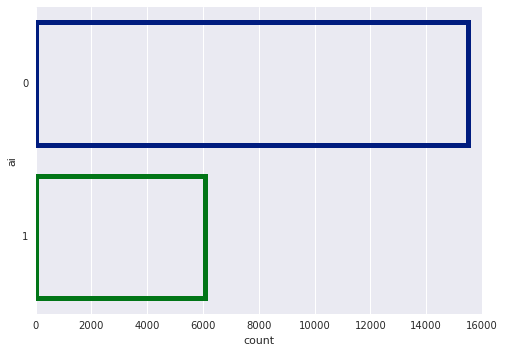In [ ]:
# Plot results for Data Management
ax= sns.countplot(y="dm", data=aq, facecolor=(0, 0, 0, 0),
linewidth=5,
edgecolor=sns.color_palette("dark", 3))

/dataquest/system/env/python3/lib/python3.4/site-packages/seaborn/categorical.py:1428: FutureWarning:

remove_na is deprecated and is a private function. Do not use.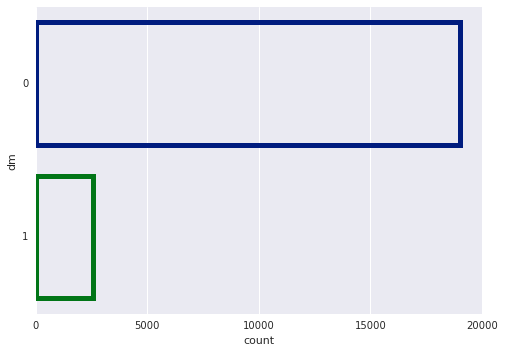In [ ]:
# Plot results from Statistics
ax=sns.countplot(y="stat", data=aq, facecolor=(0, 0, 0, 0),
linewidth=5,
edgecolor=sns.color_palette("dark", 3))

/dataquest/system/env/python3/lib/python3.4/site-packages/seaborn/categorical.py:1428: FutureWarning:

remove_na is deprecated and is a private function. Do not use.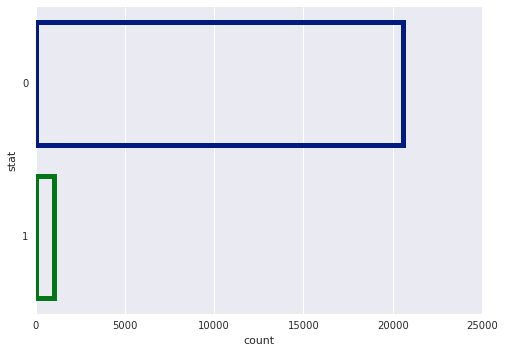### Exploring Time-Series for all Disciplines¶

From above it can be seen that AI and its components machine-learning and subfield deep-learning form the major thrust of questions at SEDS. However, other disciplines are not insignificant, and We will explore them further as a time series.

• We have already classified all ai questions as deep-learning questions. We will now use the dataframe aq to:

• Count how many questions per discipline including deep-learning are asked per time period.

• The total amount of questions per time period.
• How many deep learning and other disciplines' questions there are relative to the total amount of questions per time period.

### Analysis Strategy¶

• We will change CreationDate to string in yymm format
• Define a function get_qtr which uses string functions to extract quarters from string in format yyQn, where n is the quarter number
• apply function get_qtr to CreationDate and convert it to yyQn format
• groupby yyQn and aggregate count for all disciplines ai, dm and stat
• Plot results
In [ ]:
# change "CreationDate" to string
aq_ts = aq
aq_ts["qtr"] = aq_ts["CreationDate"].astype(str).str[2:7].str.replace('-','')
aq_ts.drop(labels="CreationDate", axis=1, inplace=True)

In [ ]:
aq_ts=aq_ts.sort_values(by='qtr', ascending=True)
print(aq_ts.head())

      Id                                               Tags  dm  stat  ai  \
496  134  {apache-hadoop, scalability, mongodb, map-reduce}   0     0   1
81    69                                       {processing}   0     0   0
80    61            {machine-learning, predictive-modeling}   0     0   0
79    59                                 {apache-hadoop, r}   0     0   0
78    52                                 {r, data-cleaning}   0     0   0

qtr
496  1405
81   1405
80   1405
79   1405
78   1405

In [ ]:
# Define function get_qtr
""" extracts quarters from yymm"""
def get_qtr(string):
year = int(string[0:2])*100
month = int(string)-year
qtr = int(((month)-1)/3)+1
return '{y}Q{q}'.format(y=string[0:2], q=qtr)

In [ ]:
# apply 'get_qtr' to 'aq_ts["qtr"]'
aq_ts["qtr"] = aq_ts["qtr"].apply(get_qtr)

In [ ]:
print(aq_ts.tail())

          Id                                               Tags  dm  stat  ai  \
21229  66591                     {optimization, neural-network}   0     0   0
21228  66590                        {visualization, r, ggplot2}   0     0   0
21227  66588                              {markov-hidden-model}   0     0   0
21240  66619  {scikit-learn, python, dataset, linear-regress...   0     0   0
21195  66530                                           {pandas}   0     0   0

qtr
21229  20Q1
21228  20Q1
21227  20Q1
21240  20Q1
21195  20Q1

In [ ]:
import numpy as np
aq_ts_pt = aq_ts.pivot_table(values = ["ai","dm","stat"], index = "qtr", aggfunc=(np.sum))

In [ ]:
print(aq_ts_pt)

       ai   dm  stat
qtr
14Q2   51   11    20
14Q3   34   11     9
14Q4   28    9     6
15Q1   26   16     6
15Q2   57   24    12
15Q3   48   17    16
15Q4   73   23    13
16Q1  120   42    21
16Q2  132   47    19
16Q3  125   41    32
16Q4  124   43    33
17Q1  167   85    33
17Q2  168   63    39
17Q3  171   79    28
17Q4  235   89    42
18Q1  334  166    43
18Q2  402  178    83
18Q3  423  182    63
18Q4  368  155    62
19Q1  606  262   100
19Q2  770  329   119
19Q3  750  315   102
19Q4  722  323    88
20Q1  155   63    18

In [ ]:
aq_ts_pt.drop(labels="20Q1", inplace=True)

In [ ]:
# Comparative Time Series Curves for "all disciplines"

fig, axes = plt.subplots()
fig.set_size_inches((14, 6))
#log scaling on x axis due to very large numbers
aq_ts_pt["ai"].plot(kind="line", c='orange', subplots=True, label="ai", legend=True)
aq_ts_pt["dm"].plot(kind="line", c='green', subplots=True, label="dm", legend=True)
aq_ts_pt["stat"].plot(kind="line", subplots=True, label="stat", legend=True)

Out[ ]:
array([<matplotlib.axes._subplots.AxesSubplot object at 0x7f17f31edeb8>],
dtype=object)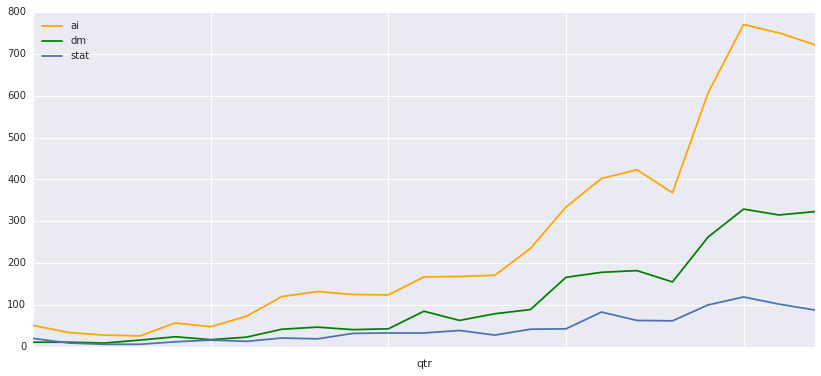In [ ]:
# Plotting Time Series: "AI Growth"

fig, axes = plt.subplots()
fig.set_size_inches((14, 6))
plt.ylim(0, 800)

aq_ts_pt["ai"].plot(kind="bar", subplots=True, label="ai", legend=True, title = "AI Growth - Time Series")
aq_ts_pt["ai"].plot(kind="line", c='orange', subplots=True, label="dm", legend=True)

Out[ ]:
array([<matplotlib.axes._subplots.AxesSubplot object at 0x7f17e6042c88>],
dtype=object)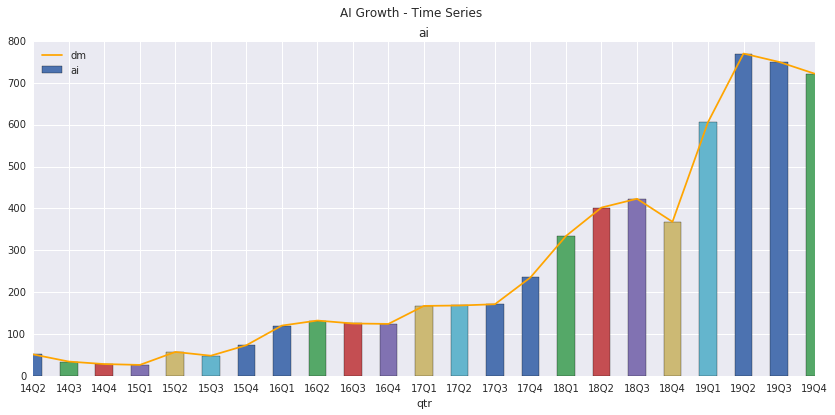In [ ]:
# Plotting Time Series: "DM Growth"

fig, axes = plt.subplots()
fig.set_size_inches((14, 6))
plt.ylim(0, 800)
aq_ts_pt["dm"].plot(kind="bar", color='orange', subplots=True, label="dm", legend=True, title = "DM Growth - Time Series")
aq_ts_pt["dm"].plot(kind="line", color='blue', subplots=True, label="dm", legend=True)

Out[ ]:
array([<matplotlib.axes._subplots.AxesSubplot object at 0x7f17e61ea780>],
dtype=object)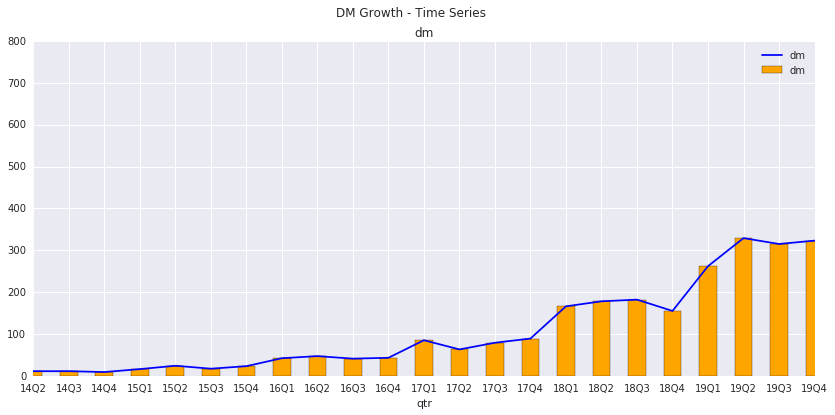In [ ]:
# Plotting Time Series: "Stat Growth"

fig, axes = plt.subplots()
fig.set_size_inches((14, 6))
plt.ylim(0, 800)
aq_ts_pt["stat"].plot(kind="bar", color='red', subplots=True, label="dm", legend=True, title = "Stat Growth - Time Series")
aq_ts_pt["stat"].plot(kind="line", color='green', subplots=True, label="dm", legend=True)

Out[ ]:
array([<matplotlib.axes._subplots.AxesSubplot object at 0x7f17e5e78da0>],
dtype=object)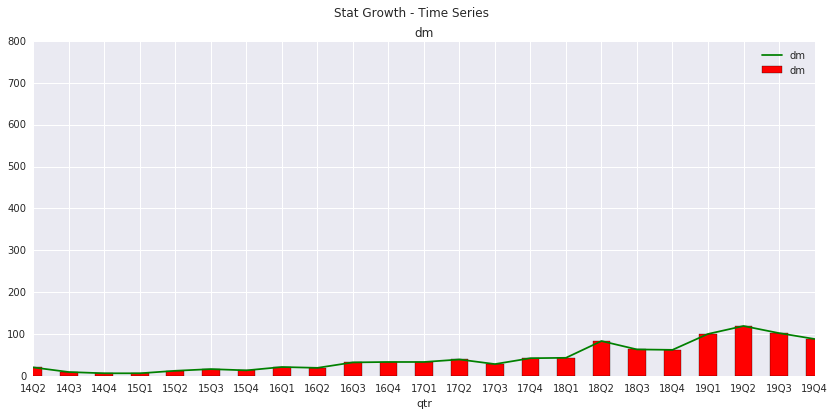### Recommendations¶

• It is clear from above that the AI subfields including deep-learning and to an extent data-management subfields have shown marked growth since 4th quarter of 2018. Perhaps, this is indicative of the interest in the field in general. A major AI discipline maths-statistics has not registered much interset despite being a major requirement for data analysis. Perhaps, it is due to requirement of STEM education in this field.

• Our content, for present, should be focused on deep-learning and data-management.